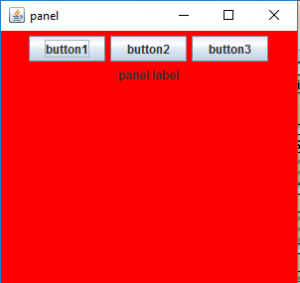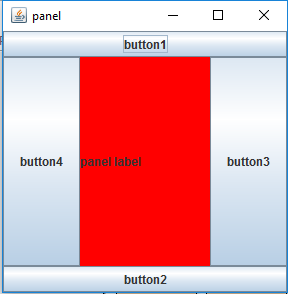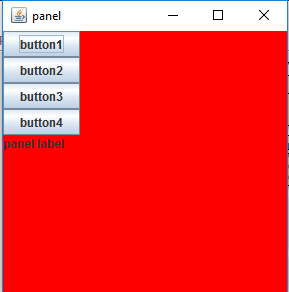Open in App
Not now

# Java Swing – JPanel With Examples

• Difficulty Level : Easy
• Last Updated : 10 Nov, 2021

JPanel, a part of the Java Swing package, is a container that can store a group of components. The main task of JPanel is to organize components, various layouts can be set in JPanel which provide better organization of components, however, it does not have a title bar.

### Constructors of JPanel

1. JPanel(): creates a new panel with a flow layout
2. JPanel(LayoutManager l): creates a new JPanel with specified layoutManager
3. JPanel(boolean isDoubleBuffered): creates a new JPanel with a specified buffering strategy
4. JPanel(LayoutManager l, boolean isDoubleBuffered): creates a new JPanel with specified layoutManager and a specified buffering strategy

### Commonly used Functions of JPanel

2. setLayout(LayoutManager l): sets the layout of the container to the specified layout manager
3. updateUI(): resets the UI property with a value from the current look and feel.
4. setUI(PanelUI ui): sets the look and feel of an object that renders this component.
5. getUI(): returns the look and feel object that renders this component.
6. paramString(): returns a string representation of this JPanel.
7. getUIClassID(): returns the name of the Look and feel class that renders this component.
8. getAccessibleContext(): gets the AccessibleContext associated with this JPanel.

Let us take a sample program in order to illustrate the use of JPanel class by appending sequential execution snapshots of outputs justifying the below program sets as follows:

Example:

## Java

 `// Java Program to Create a Simple JPanel ` `// and Adding Components to it ` ` `  `// Importing required classes ` `import` `java.awt.*; ` `import` `java.awt.event.*; ` `import` `javax.swing.*; ` ` `  `// Class 1 ` `// Helper class extending JFrame class ` `class` `solution ``extends` `JFrame { ` ` `  `    ``// JFrame ` `    ``static` `JFrame f; ` ` `  `    ``// JButton ` `    ``static` `JButton b, b1, b2; ` ` `  `    ``// Label to display text ` `    ``static` `JLabel l; ` ` `  `    ``// Main class ` `    ``public` `static` `void` `main(String[] args) ` `    ``{ ` `        ``// Creating a new frame to store text field and ` `        ``// button ` `        ``f = ``new` `JFrame(``"panel"``); ` ` `  `        ``// Creating a label to display text ` `        ``l = ``new` `JLabel(``"panel label"``); ` ` `  `        ``// Creating a new buttons ` `        ``b = ``new` `JButton(``"button1"``); ` `        ``b1 = ``new` `JButton(``"button2"``); ` `        ``b2 = ``new` `JButton(``"button3"``); ` ` `  `        ``// Creating a panel to add buttons ` `        ``JPanel p = ``new` `JPanel(); ` ` `  `        ``// Adding buttons and textfield to panel ` `        ``// using add() method ` `        ``p.add(b); ` `        ``p.add(b1); ` `        ``p.add(b2); ` `        ``p.add(l); ` ` `  `        ``// setbackground of panel ` `        ``p.setBackground(Color.red); ` ` `  `        ``// Adding panel to frame ` `        ``f.add(p); ` ` `  `        ``// Setting the size of frame ` `        ``f.setSize(``300``, ``300``); ` ` `  `        ``f.show(); ` `    ``} ` `}`

Output:Example 2:

## Java

 `// Java Program to Create a JPanel with a Border Layout ` `// and Adding Components to It ` ` `  `// Importing required classes ` `import` `java.awt.*; ` `import` `java.awt.event.*; ` `import` `javax.swing.*; ` ` `  `// Main class ` `// Extending JFrame class ` `class` `solution ``extends` `JFrame { ` ` `  `    ``// JFrame ` `    ``static` `JFrame f; ` ` `  `    ``// JButton ` `    ``static` `JButton b, b1, b2, b3; ` ` `  `    ``// Label to display text ` `    ``static` `JLabel l; ` ` `  `    ``// Main driver method ` `    ``public` `static` `void` `main(String[] args) ` `    ``{ ` `        ``// Creating a new frame to store text field and ` `        ``// button ` `        ``f = ``new` `JFrame(``"panel"``); ` ` `  `        ``// Creating a label to display text ` `        ``l = ``new` `JLabel(``"panel label"``); ` ` `  `        ``// Creating a new buttons ` `        ``b = ``new` `JButton(``"button1"``); ` `        ``b1 = ``new` `JButton(``"button2"``); ` `        ``b2 = ``new` `JButton(``"button3"``); ` `        ``b3 = ``new` `JButton(``"button4"``); ` ` `  `        ``// Creating a panel to add buttons ` `        ``// and a specific layout ` `        ``JPanel p = ``new` `JPanel(``new` `BorderLayout()); ` ` `  `        ``// Adding buttons and textfield to panel ` `        ``// using add() method ` `        ``p.add(b, BorderLayout.NORTH); ` `        ``p.add(b1, BorderLayout.SOUTH); ` `        ``p.add(b2, BorderLayout.EAST); ` `        ``p.add(b3, BorderLayout.WEST); ` `        ``p.add(l, BorderLayout.CENTER); ` ` `  `        ``// setbackground of panel ` `        ``p.setBackground(Color.red); ` ` `  `        ``// Adding panel to frame ` `        ``f.add(p); ` ` `  `        ``// Setting the size of frame ` `        ``f.setSize(``300``, ``300``); ` ` `  `        ``f.show(); ` `    ``} ` `}`

Output:Example 3:

## Java

 `// Java Program to Create a JPanel with a Box layout ` `// and Adding components to it ` ` `  `// Importing required classes ` `import` `java.awt.*; ` `import` `java.awt.event.*; ` `import` `javax.swing.*; ` ` `  `// Main class ` `// Extending JFrame class ` `class` `solution ``extends` `JFrame { ` ` `  `    ``// JFrame ` `    ``static` `JFrame f; ` ` `  `    ``// JButton ` `    ``static` `JButton b, b1, b2, b3; ` ` `  `    ``// Label to display text ` `    ``static` `JLabel l; ` ` `  `    ``// Main drive method ` `    ``public` `static` `void` `main(String[] args) ` `    ``{ ` `        ``// Creating a new frame to store text field and ` `        ``// button ` `        ``f = ``new` `JFrame(``"panel"``); ` ` `  `        ``// Creating a label to display text ` `        ``l = ``new` `JLabel(``"panel label"``); ` ` `  `        ``// Creating a new buttons ` `        ``b = ``new` `JButton(``"button1"``); ` `        ``b1 = ``new` `JButton(``"button2"``); ` `        ``b2 = ``new` `JButton(``"button3"``); ` `        ``b3 = ``new` `JButton(``"button4"``); ` ` `  `        ``// Creating a panel to add buttons and ` `        ``// textfield and a layout ` `        ``JPanel p = ``new` `JPanel(); ` ` `  `        ``// Setting box layout ` `        ``p.setLayout(``new` `BoxLayout(p, BoxLayout.Y_AXIS)); ` ` `  `        ``// Adding buttons and textfield to panel ` `        ``p.add(b); ` `        ``p.add(b1); ` `        ``p.add(b2); ` `        ``p.add(b3); ` `        ``p.add(l); ` ` `  `        ``// Setting background of panel ` `        ``p.setBackground(Color.red); ` ` `  `        ``// Adding panel to frame ` `        ``f.add(p); ` ` `  `        ``// Setting the size of frame ` `        ``f.setSize(``300``, ``300``); ` ` `  `        ``f.show(); ` `    ``} ` `}`

Output:Henceforth, we are successfully able to generate buttons in our panel.

Note: In the previous Program, border layout and Box Layout are used. Different other layouts can be used to organize the components in a definite pattern, such as card layout, grid layout, etc.

My Personal Notes arrow_drop_up
Related Articles